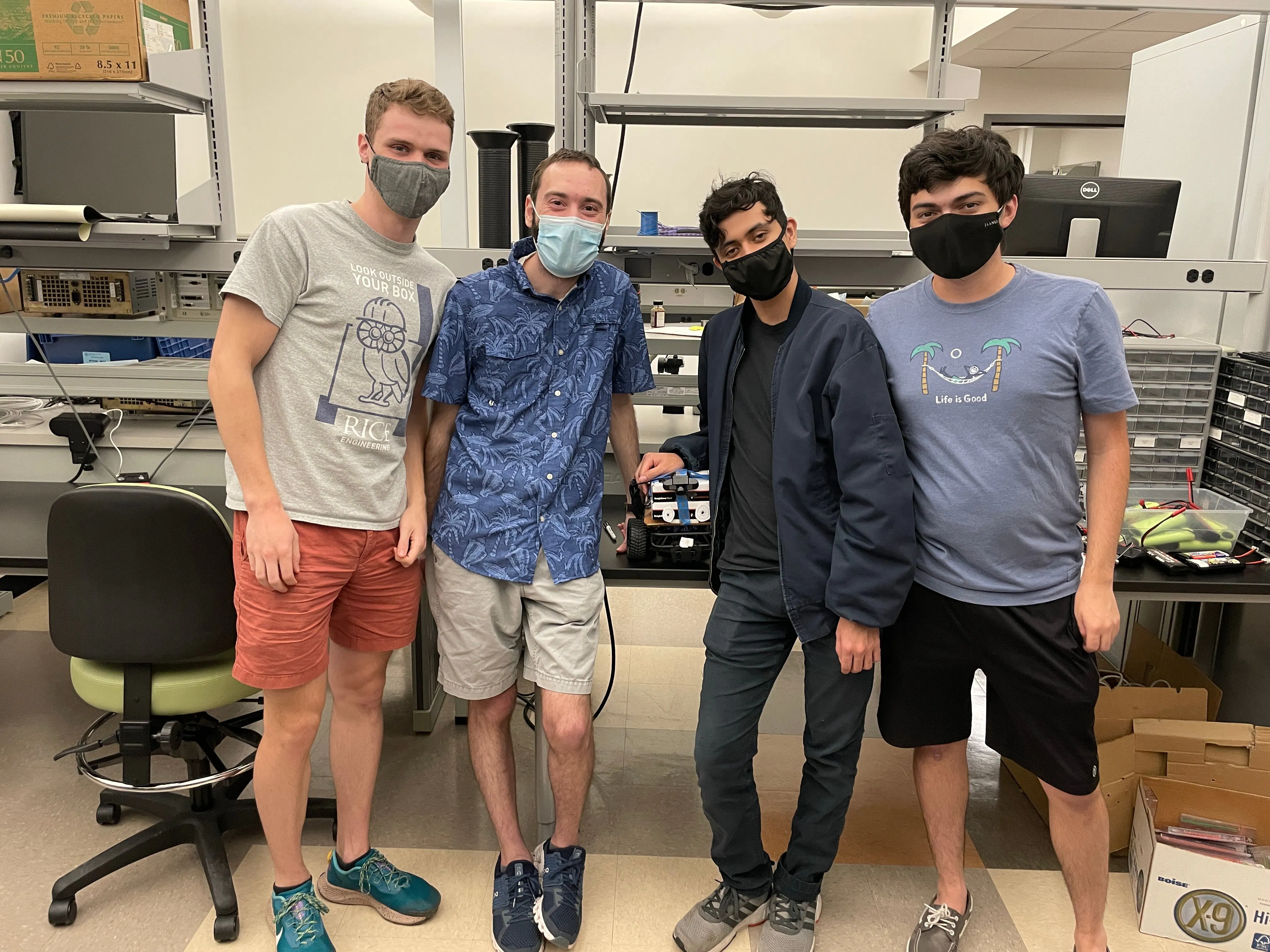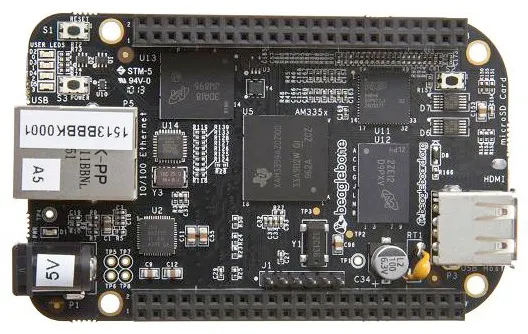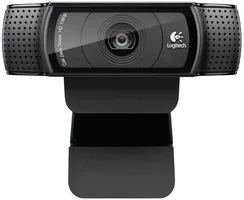# Rockee

We teach a car to stay in it's lane and stop on a dime

IntermediateShowcase (no instructions)4 hours171## Things used in this project

### Hardware componentsBeagleBoard.org BeagleBone Black
×1Webcam, Logitech® HD Pro
×1
×1
 usb hub
×1

### Software apps and online servicesOpenCV

## Code

### driver.py

Python
This contains the lane keeping and stopping logic
```import cv2
import numpy as np
import math

# This function filters out all non blue pixels and applys a canny edge detection
# filter to the resulting image
def detect_edges(frame):
# lower limit of blue color
lower_blue = np.array([90, 120, 0], dtype="uint8")
# upper limit of blue color
upper_blue = np.array([150, 255, 255], dtype="uint8")
# this mask will filter out everything but blue
# run canny edge detection
return edges

# The average value of red pixels that must be reached to stop
STOP_THRESHOLD = 15.0

# This function filters out all non red pixels, and averages the resulting image to quantify the
# amount of red in the frame. Returns true if the threshold to detect a stop sign is reached.
def detect_stop_sign(frame):
# lower red limit
lower_red = np.array([160, 100, 0], dtype="uint8")
# upper red limit
upper_red = np.array([180, 255, 255], dtype="uint8")

# filter out all non read pixels
red_stuff = cv2.inRange(frame, lower_red, upper_red)
# take average of red pixels
val = np.mean(red_stuff)
return val > STOP_THRESHOLD

# This function crops the image to just the region of interest.
def region_of_interest(edges):
height, width = edges.shape  # extract the height and width of the edges frame
# make an empty matrix with same dimensions of the edges frame

# only focus lower half of the screen
# specify the coordinates of 4 points (lower left, upper left, upper right, lower right)
polygon = np.array([[
(0, height),
(0,  height/2),
(width, height/2),
(width, height),
]], np.int32)

cv2.fillPoly(mask, polygon, 255)  # fill the polygon with blue color
return cropped_edges

# This function performs the hough transform on the image to detect the line segments in the image.
# The input should be the image after the edge detection and roi functions are called.
def detect_line_segments(cropped_edges):
rho = 1
theta = np.pi / 180
min_threshold = 10
# perform hough transform
line_segments = cv2.HoughLinesP(cropped_edges, rho, theta, min_threshold,
np.array([]), minLineLength=5, maxLineGap=0)
return line_segments

# This is a helper function to make points that represent a given line in the image
def make_points(frame, line):
height, width, _ = frame.shape
slope, intercept = line
y1 = height  # bottom of the frame
y2 = int(y1 / 2)  # make points from middle of the frame down

if slope == 0:
slope = 0.1

x1 = int((y1 - intercept) / slope)
x2 = int((y2 - intercept) / slope)

return [[x1, y1, x2, y2]]

# This function groups the lines detected in the image into those for the left and right lane and
# averages them to get a single line for each edge
def average_slope_intercept(frame, line_segments):
lane_lines = []

if line_segments is None:
print("no line segment detected")
return lane_lines

height, width, _ = frame.shape
left_fit = []
right_fit = []
boundary = 1/3

# set region that corresponds to lines in the left edge
left_region_boundary = width * (1 - boundary)
# set region that corresponds to lines in the right edge
right_region_boundary = width * boundary

for line_segment in line_segments:
for x1, y1, x2, y2 in line_segment:
if x1 == x2:
# skip vertical lines, slope = infinity
continue
# calculate slope
slope = (y2 - y1) / (x2 - x1)
# calculate intercept
intercept = y1 - (slope * x1)

# add edge to left or right lane lines depeding on the location of the line
# in the frame
if slope < 0 and x1 < left_region_boundary and x2 < left_region_boundary:
left_fit.append((slope, intercept))
elif x1 > right_region_boundary and x2 > right_region_boundary:
right_fit.append((slope, intercept))

# average the left lane lines
if len(left_fit) > 0:
left_fit_average = np.average(left_fit, axis=0)
lane_lines.append(make_points(frame, left_fit_average))
# average the right lane lines
if len(right_fit) > 0:
right_fit_average = np.average(right_fit, axis=0)
lane_lines.append(make_points(frame, right_fit_average))

# lane_lines is a 2-D array consisting the coordinates of the right and left lane lines
# for example: lane_lines = [[x1,y1,x2,y2],[x1,y1,x2,y2]]
# where the left array is for left lane and the right array is for right lane
# all coordinate points are in pixels
return lane_lines

# This function overlays the lane lines on the image
def display_lines(frame, lines, line_color=(0, 255, 0), line_width=6):  # line color (B,G,R)
line_image = np.zeros_like(frame)

# Create the lines
if lines is not None:
for line in lines:
for x1, y1, x2, y2 in line:
cv2.line(line_image, (x1, y1), (x2, y2),
line_color, line_width)

# add the lines to the image
line_image = cv2.addWeighted(frame, 0.8, line_image, 1, 1)
return line_image

# This function takes in the lane lines and computes the average to find the heading angle for the car.
# Returns the angle (in degrees 0..180) that the car should turn
def get_steering_angle(frame, lane_lines):
height, width, _ = frame.shape

if len(lane_lines) == 2:  # if two lane lines are detected
# extract left x2 from lane_lines array
_, _, left_x2, _ = lane_lines
# extract right x2 from lane_lines array
_, _, right_x2, _ = lane_lines
mid = int(width / 2)
x_offset = (left_x2 + right_x2) / 2 - mid
y_offset = int(height / 2)

elif len(lane_lines) == 1:  # if only one line is detected
x1, _, x2, _ = lane_lines
x_offset = x2 - x1
y_offset = int(height / 2)

elif len(lane_lines) == 0:  # if no line is detected
x_offset = 0
y_offset = int(height / 2)

# Compute the final angle
angle_to_mid_deg = int(angle_to_mid_radian * 180.0 / math.pi)
steering_angle = angle_to_mid_deg + 90

return steering_angle

# This function takes in the new heading angle and overlays the resulting heading line on the image.
def display_heading_line(frame, steering_angle, line_color=(0, 0, 255), line_width=5):

height, width, _ = frame.shape

steering_angle_radian = steering_angle / 180.0 * math.pi
x1 = int(width / 2)
y1 = height
x2 = int(x1 - height / 2 / math.tan(steering_angle_radian))
y2 = int(height / 2)
# create the line
cv2.line(heading_image, (x1, y1), (x2, y2), line_color, line_width)
# overlay the line on the image

# This function sets the speed by changing the PWM value for the pin controlling the motor
def set_drive_speed(speed):
PWM.start("P9_14", speed, 50)

# This function sets the turning angle by changing the PWM value for the pin controlling the servo motor
def set_turn_angle(angle):
PWM.start("P8_13", angle, 50)

# This represents the default PWM value for 'straight' in the servo motor.
# It is not 7.5 to counteract slight skew in the wheels
DEFAULT_ANGLE = 7.0

# Steers the car based on a heading angle. If the angle is within +/- linear range from 0,
# then it scales the PWM value linearly within this range s.t. the change in PWM is 0 at 0 degrees, and
# max_delta at +/- linear range degrees. Outside of the linear_range degrees it defaults to the DEGAULT_ANGLE +/- max_delta
def steer(steering_angle, linear_range, max_delta):
deviation = steering_angle - 90
heading = DEFAULT_ANGLE - (deviation * (max_delta/linear_range))

# Restrict the range of the resulting PWM value

# log the deviation and heading

# Optional delta to increase speed by after stopping to counteract less acceleration
SPEED_DELTA = 0.0

# This class controls the car
class Driver:
def __init__(self):
# set the default PWM values in the pins
set_drive_speed(7.5)
set_turn_angle(7.5)

# This function runs the car and performs lane keeping and stopping.
def run(self, speed, linear_range, max_delta, show_image=False):
# Capture the video
self.video = cv2.VideoCapture(0)
# scale the video feed
self.video.set(cv2.CAP_PROP_FRAME_WIDTH, 320)
self.video.set(cv2.CAP_PROP_FRAME_HEIGHT, 240)
# Start the motor
set_drive_speed(speed)
try:
# indicates if the previous frame was at a stop sign. This is to ensure the car doesn't stop multiple times
# at consecutive frames at a stop sign
last_stop = False
count = 401
has_stopped_before = False
while True:
if count == 30:
# How many frames to wait for after stopping. After 30 frames
# restart the motor
set_drive_speed(speed+SPEED_DELTA)
count += 1 # count frames

# get image from camera
# resize image to speedup processing
frame = cv2.resize(frame, (160, 120))
# covert to hsv
hsv = cv2.cvtColor(frame, cv2.COLOR_BGR2HSV)

if detect_stop_sign(hsv):
# If stop sign is detected and wasn't detected in previous frame then stop
if not last_stop:
set_drive_speed(7.5)
print("STOPPING")
last_stop = True
count = 0
if has_stopped_before:
# If the car has stopped before then it is at the final stop sign and it can end the
# control loop
self.release()
print("Finished")
break
has_stopped_before = True
else:
# If no stop sign is detected mark that it has left the stop sign
last_stop = False

# detect edges
edges = detect_edges(hsv)

# get region of interest
roi = region_of_interest(edges)

# detect all lane edge lines
lines = detect_line_segments(roi)
# average egde lines to get lane lines
lane_lines = average_slope_intercept(frame, lines)
# get the steering angle
steering_angle = get_steering_angle(frame, lane_lines)
# steer the car based on the angle
steer(steering_angle, linear_range, max_delta)

# display the heading lines and edge lines
output = display_lines(frame, lane_lines)

if show_image:
# optionally display the image. This is optional because
# this can slow down the processing
cv2.imshow('view', output)
key = cv2.waitKey(1)
if key == 27:
break
# if control-c is hit then stop the car
except KeyboardInterrupt:
self.release()
print("Stopped")

# stops the car and resets PWM values and releases video.
def release(self):
self.video.release()
cv2.destroyAllWindows()
set_drive_speed(7.5)
set_turn_angle(7.5)
```

## Credits

### mac28

1 project • 0 followers

### Michael Angino

1 project • 0 followers

### Kevin Lata

1 project • 0 followers

### Nicholas Meisburger

1 project • 0 followers